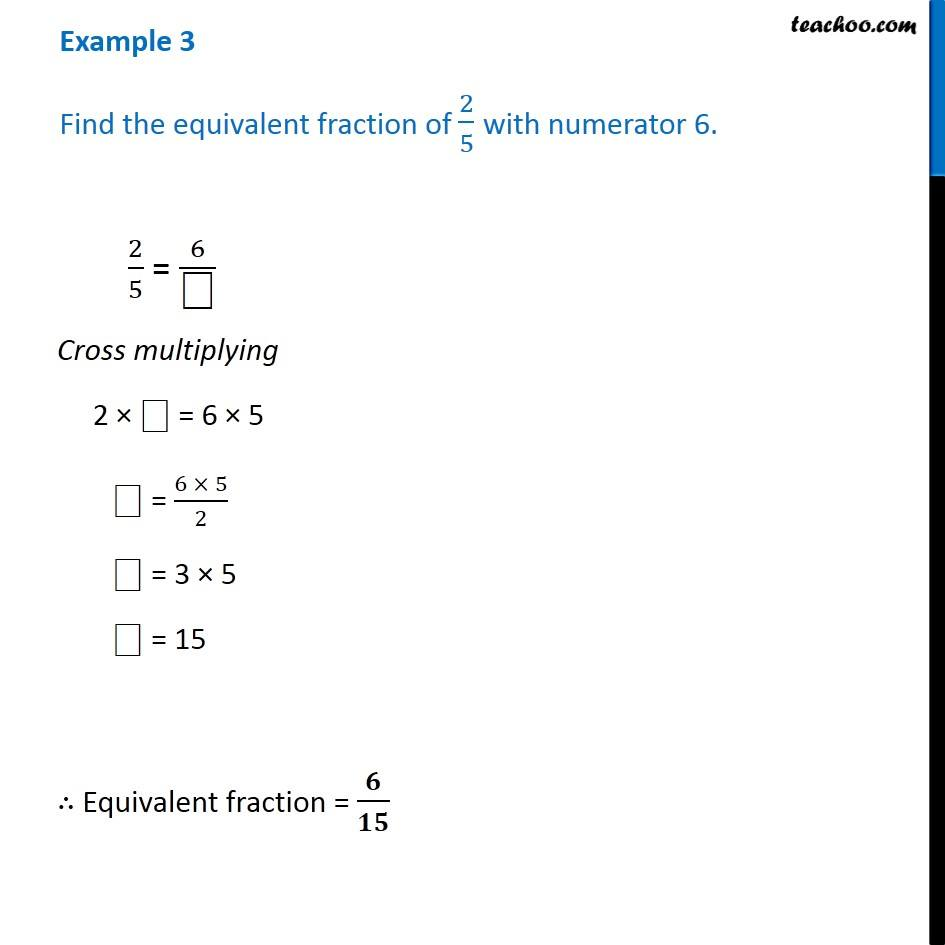Examples

Chapter 7 Class 6 Fractions
Serial order wiseGet live Maths 1-on-1 Classs - Class 6 to 12

### Transcript

Example 3 Find the equivalent fraction of 2/5 with numerator 6. 2/5 = 6/" " Cross multiplying 2 " " = 6 5 " " = (6 5)/2 " " = 3 5 " " = 15 Equivalent fraction = /